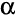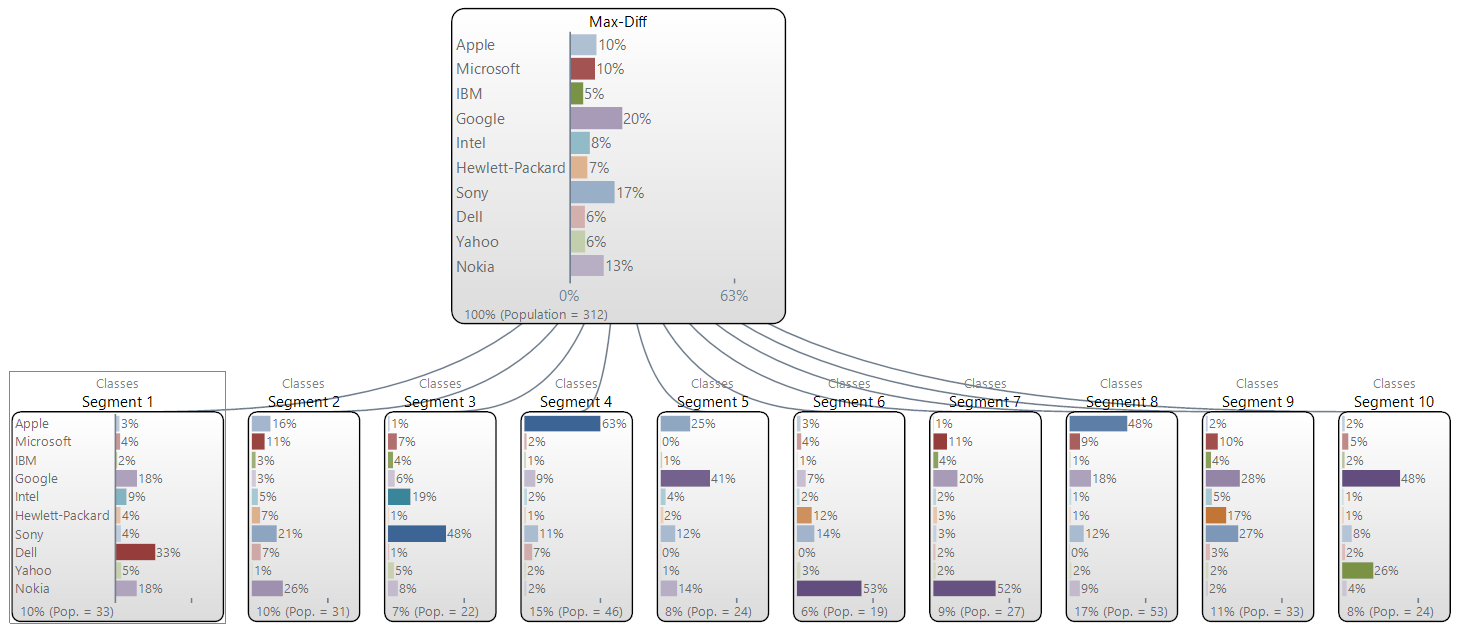# MaxDiff Case Study

This page describes the 'exotic' approach to analyzing MaxDiff experiments in Q. Refer to MaxDiff on the Displayr wiki for a more general introduction and links to key resources.

## Setting up the data

In general, the most straightforward approach for setting up a MaxDiff experiment is using Create > Marketing > MaxDiff > Analyze as a Ranking Question > MaxDiff Setup from an Experimental Design. However, in this case study, you need to instead manually set the Question Type:

1. Download the example data file, File:ItMaxDiff.sav, which has been created in accordance with the MaxDiff Specifications.
2. Import it into Q.
3. Go to the Variables and Questions tab.
4. Select the relevant rows (in this case, rows 6 to 65).
5. Right-click and select Set Question.
6. Enter a desired name (e.g., MaxDiff).
7. Set the Question Type to Ranking.
8. Press OK.
9. Double click on any one of the row numbers so that the question is in a SUMMARY tables on the Outputs Tab.

## Interpreting a MaxDiff SUMMARY table

The following table shows the default statistics for a MaxDiff SUMMARY table. A rank-ordered logit model with ties has been automatically estimated and the percentages shown are estimates of preference share. This example is from a MaxDiff study on technology brands and the interpretation of this table is that if respondents had been presented with all ten brands it is estimated that 10% would have selected Apple, 10% Microsoft, 5% IBM, etc.

By right-clicking on the table and selecting Statistics - Cells we can select additional statistics, including the computed Coefficients and t-Statistics. To obtain a more complete set of diagnostics, select all the cells on the table and press(Planned Tests Of Statistical Significance), which will obtain outputs like those below. Note that:

• These diagnostics are provided for statisticians; it is not essential that you understand these in order to intepret the MaxDiff study.
• The S.E. is the Standard Error of the Probability % that is seen on the table displayed on the Outputs Tab, not Coef. Thus, the p-Values and t-Stats shown below are not computed by dividing the coefficient by the standard error. Instead, the tests show the differences relative to the average Probability %.

## Crosstabs of MaxDiff data

Create a crosstab by selecting Q1_Gender in the Brown Drop-down Menu. When a MaxDiff question is selected in the Blue Drop-down Menu and a Pick One (i.e., categorical) question is selected in the Brown Drop-down Menu Q automatically re-estimates the model for each of the categories of the question in the columns of the table and automatically tests for difference between the columns. Thus, looking at the example below, we can see the most preferred brand, Google, is equally preferred by the genders, whereas Apple is more preferred among women (11% versus 9% for men), while Intel and HP are stronger among the men.

Note that by re-estimating the model within each sub-group the resulting statistical tests have substantially more power than those that are conducted when testing using Individual-Level Parameters.[note 1]

## Latent class models

Latent class analysis can be used to identify groups of consumers that exhibit similar preferences in a MaxDiff experiment. A latent class model is estimated as follows:

1. Select Create > Segments. In this example, ensure that MaxDiff is selected.
2. Ensure that the MaxDiff question is selected in Questions to analyze and that Form segments by is set to splitting by individuals (latent class analysis, cluster analysis, mixture models) (in this case study, it will be set by default to splitting by questions (tree) due to gender being selected in the Brown Drop-down Menu.
3. If desired, specify the number of segments to be computed (by default, this is automatically selected using the Bayesian Information Criterion).
4. Press OK.

### Latent class outputs

#### Tree

The first output generated by the latent class analysis is a technical report (described in the next section), but the main output is a 'tree', like the one shown below. The 'node' at the top of the screen shows the results for the total sample. Each of the nodes underneath show the results for the specific segments (10 in this example), with the sizes of the segments shown at the bottom.#### Grow Settings and Analysis Report

Refer to Latent Class Analysis for a general discussion of these outputs. The discussion below concentrates upon the outputs most relevant for MaxDiff.

##### Class Sizes

The class sizes are estimates of the proportion of people in different segments. In this example we can see that ten segments (i.e., groups of people with different preferences) have been identified with estimated sizes from 6% to 17%. Note that these are estimates of the population and generally the proportion of respondents in a survey that are assigned to each of these clusters will differ somewhat from these estimated numbers (due to the inherent uncertainty about how people are allocated into segments). They may further differ from the sample size due to weighting.

##### Class Parameters

The Probabilities are the Probability %s discussed above. The Parameters are the Coefficients described above. Please note that the probabilities are the same as those shown on the 'tree', but will differ from any created from a crosstab of the latent class analysis with the MaxDiff question (this is because the segment membership variable is computed by allocating each person to one and only one class and thus it contains a degree of error, because generally there is insufficient information to know with certainty which class a respondent should be allocated to).

##### Individual-level parameter shrinkage

This outputs indicates the level of inaccuracy obtained if computing individual-level parameters (see Individual-Level Parameters). The output below reveals that if using the individual-level parameters the variation observed in the data will be about 90% of the true variation.

##### Parameters

This table shows the estimated coefficients of the model and associated statistics for inference.

### Segment membership variable

When you use latent class analysis a new Pick One question (i.e., variable) is automatically created called Segments 8/05/2013 8:42:22 PM (using the date and time that you created the analysis). This can then be crosstabulated with other variables in the study (however, the statistical tests used in such analysis have less power than if crosstabbing directly with the MaxDiff question).

### Individual-level parameters

Estimates of the MaxDiff coefficients can be computed for each respondent by:

• Right-clicking on the tree and selecting Save Individual-Level Parameters Means and Standard Deviations (note that you need to specify Case IDs on the Data tab prior to selecting this option).
• Selecting RAW DATA in the Brown Drop-down Menu.

These parameters are indexed against the first brand. See Individual-Level Parameters for more information about these parameters.

## Hierarchical Bayes

Sawtooth Software markets a technique for analyzing MaxDiff that they refer to as 'hierarchical Bayes'. This model assumes a multivariate normal mixing distribution. The same theoretical model can be selected in Q by following the steps above for conducting a latent class analysis, but with the following changes: